# The Mandelbrot Set¶

#### ECE 5760, Fall 2020, Adams/Land¶

Note: Written as a supplement to Lectures 18, 19, and 20 for the Fall 2020 ECE 5760 course.

In :
from IPython.display import Image
from IPython.core.display import HTML
import plotly.graph_objects as go
%matplotlib inline
from ipywidgets import interactive
import matplotlib.pyplot as plt
import pandas as pd
import numpy
import warnings
warnings.filterwarnings('ignore')


## A demo¶

The demonstration below shows calculation/rendering of the Mandelbrot set using the DE1-SoC. Zooming/panning is done with a mouse, and the visualization toggles to Julia sets with a button press.

## The first goal¶

The Mandelbrot set is simply too interesting and too lovely to introduce as a set of equations for implementation. So, before we discuss how we'll implement this on the FPGA, I want to first attempt to explain what the Mandelbrot set is and why it's interesting. I'm going to try to do that by following a train of thought similar to that which Benoit Mandelbrot and others (including Hubbard, here at Cornell) were following when they discovered and subsequently explored and sought to understand this structure.

## Thinking about iterations . . .¶

#### The first iteration we'll consider¶

The train of thought that leads to the Mandelbrot set begins with thinking about iterations. An iteration is simply a rule which we apply over and over again. So, for example, the iteration below tells us to start with some number, $x_0$, and to square it repeatedly. If $x_0$ is 2, then $x_1$ is 4, $x_2$ is 16, etc.

\begin{align} x_{N+1} &= x_N^2 \end{align}

The question that folks were asking about these iterations was: for which choices of $x_0$ does this iteration remain stable (not go to infinity), and for which choices of $x_0$ does it go to infinity? Is it obvious that, for this iteration, any $x_0$ in the range [-1,1] is stable, and any $x_0$ outside that range is unstable? We could color the parts of the real number line which are stable as shown below.

In :
Image(filename = "line.png", width=1000, height=1000)

Out: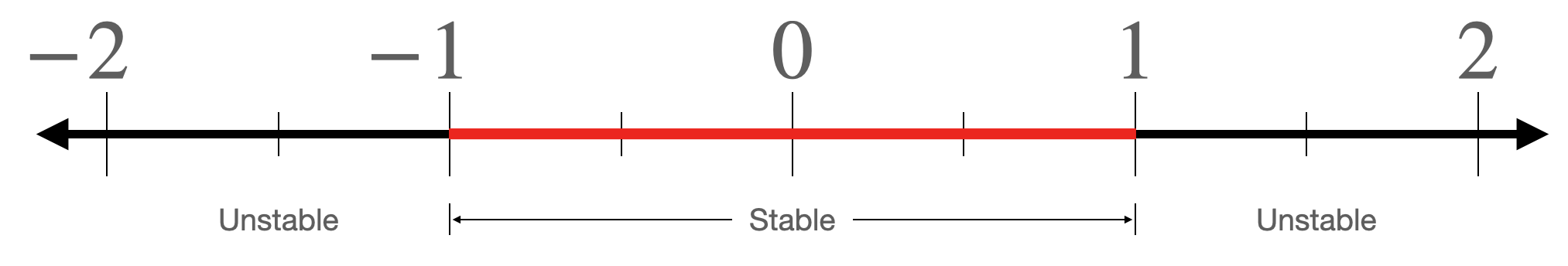#### The next iteration we'll consider¶

Let's consider almost the same iteration, except we'll allow for our numbers to be complex. Instead of representing our numbers on the real number line, we represent them in the complex plane. Our numbers can have real parts and imaginary parts.

\begin{align} z_{N+1} &= z_N^2 \end{align}

As before, the question is: for which choices of $z_0$ does this iteration remain stable (not go to infinity), and for which choices of $z_0$ does it go to infinity? As many of you will recall, when you square a complex number with magnitude less than one (i.e. a number that is less than one unit from the origin of the complex plane), the resulting complex number has a smaller magnitude. This is the complex extension of the iteration above. So, the region of stability for this iteration is a circle with radius 1 centered at the origin.

Suppose that you couldn't figure out what this region of stability looked like. One strategy that you might deploy in order to get a sense for it might be to populate the complex plane with test points, and then to run the iteration on each of those test points. If you run the iteration some large number of times (say, 1000) and it still hasn't gone above some threshold value (say, 1), then we'll assume that particular point is inside the region of stability. If it exceeds some threshold value, we'll assume it's outside the region of stability.

For example, consider the array of test points below. We'll run the above iteration on each of these test points. If the iteration diverges at that point, we'll ignore it. If it converges, we'll mark it with an x. This way, we can get a visual sense for what the region of stability looks like.

In :
x = numpy.linspace(-2, 1, 55)
y = numpy.linspace(-1, 1, 51)
X, Y = numpy.meshgrid(x, y)
plt.figure(2, figsize=(12, 8))
plt.plot(X, Y, 'b.', alpha=0.5); plt.show()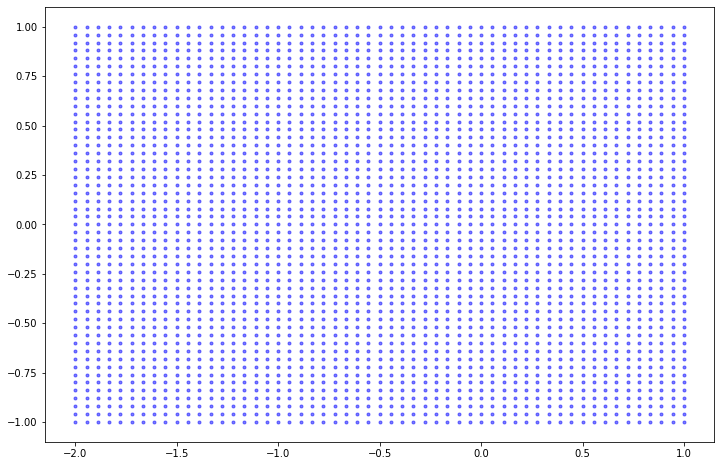Run the iteration on each point:

In :
plt.figure(2, figsize=(12, 8))
for i in x:
for j in y:
xiter = i
yiter = j
newx = xiter
newy = yiter
count = 0
while(count<1000 and (newx**2. + newy**2. < 1)):
newx = xiter**2. - yiter**2.
newy = 2*xiter*yiter
xiter = newx
yiter = newy
count += 1
if (count==1000):
plt.plot(i, j, marker='x', color='black')
else:
plt.plot(i, j, 'b.', alpha=0.1)
plt.show()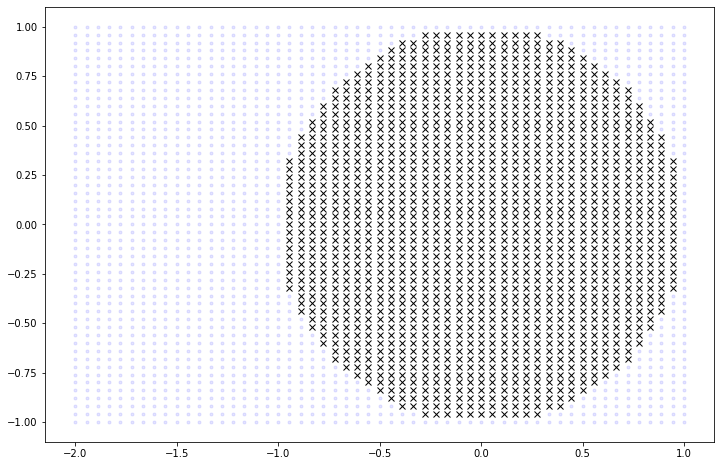As expected, we get a low resolution approximation of a circle of radius 1 centered at the origin of the complex plane.

#### One more iteration . . .¶

Let us now consider an iteration that is almost the same as the above iteration. We'll assume that $z_0$ is 0, but we'll add some complex constant $c$ every time we update the iteration.

\begin{align} z_{N+1} &= z_N^2 + c \hspace{1cm} \text{where $z_0=0$} \end{align}

Similar to before, the question is: for which choices of $c$ does this iteration remain stable (not go to infinity), and for which choices of $c$ does it go to infinity? Like we did for the previous iteration, let us populate the complex plane with test points to attempt to get an understanding for what this region of stability looks like. When we run this iteration for each test point and mark those that converge with an x, we get the following image:

In :
plt.figure(2, figsize=(12, 8))
for i in x:
for j in y:
xiter = 0
yiter = 0
newx = xiter
newy = yiter
count = 0
while(count<100 and (newx**2. + newy**2. < 4)):
newx = xiter**2. - yiter**2. + i
newy = 2*xiter*yiter + j
xiter = newx
yiter = newy
count += 1
if (count==100):
plt.plot(i, j, marker='x', color='black')
else:
plt.plot(i, j, 'b.', alpha=0.1)
plt.show()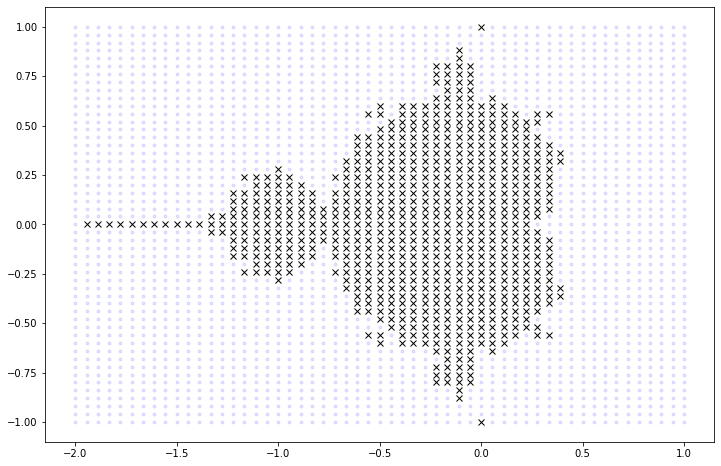What the hell is that? Benoit Mandelbrot would have asked himself the same thing when this came out of the printer in IBM. It is not obvious at this resolution what the edge of this region of convergence looks like. But, in the late 70's, this is the level of resolution that computers were capable of visualizing in reasonable amounts of time. In fact, the first ever published image of the Mandelbrot set is shown below, at approximately the same resolution as it is shown above.

In :
Image(filename = "first.png", width=500, height=500)

Out: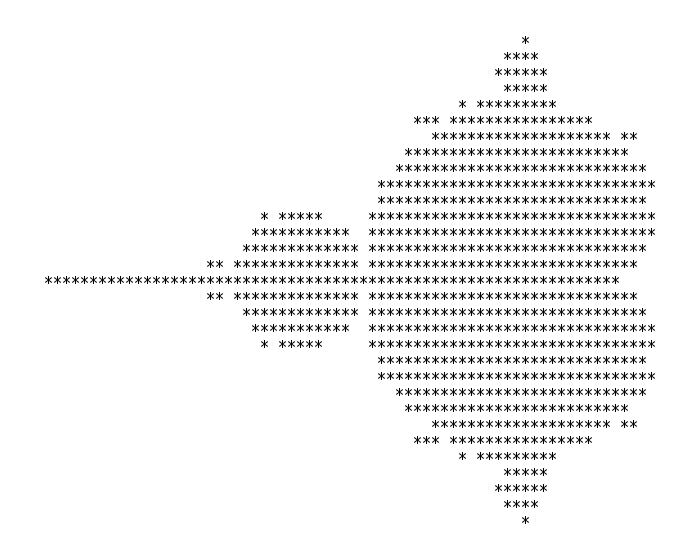As computers became more powerful, this iteration could be run on more and more test points, increasing the resolution for this region of stability. Below is the same picture, but with more test points:

In :
Image(filename = "two.png", width=500, height=500)

Out: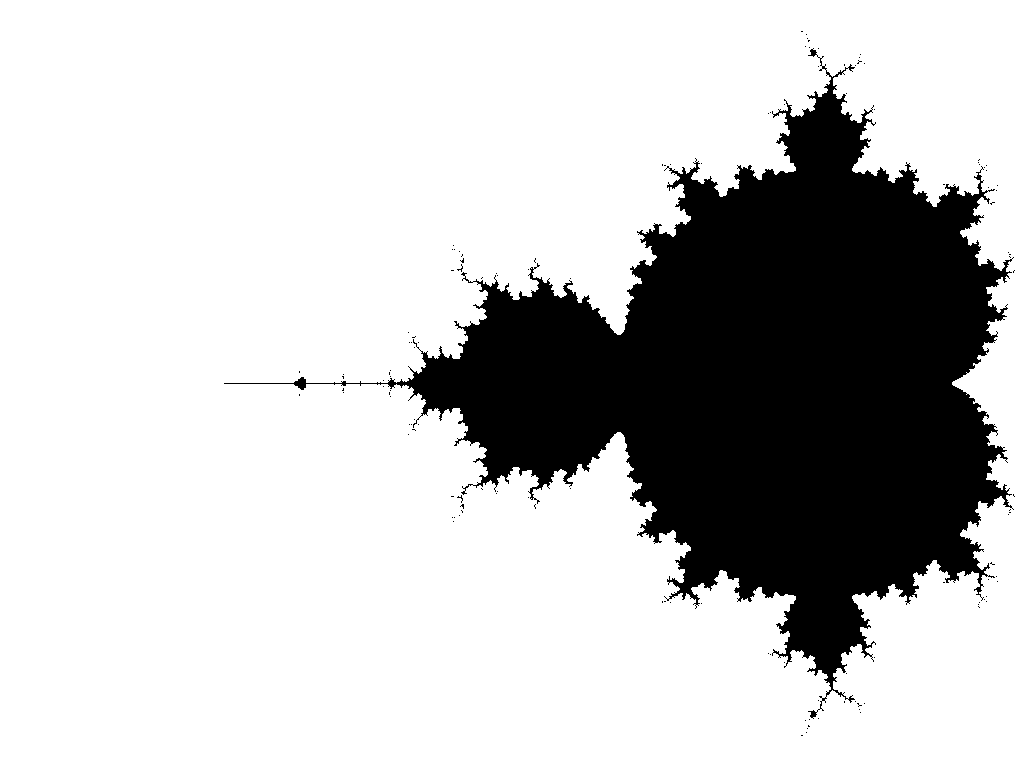Images like the one above have since become some of the most famous in mathematics. Often, you'll see them drawn not just in black and white, but with the unstable points colored in proportion to how unstable they are. (Did the point diverge in 2 iterations? If so, color it some color. Did it take 20 iterations? Color it a different color. 500 iterations? A different color again. This colorizations yield images like that shown below:

In :
Image(filename = "three.png", width=500, height=500)

Out: